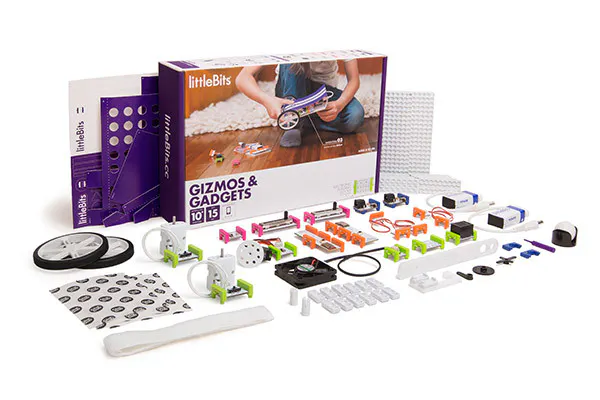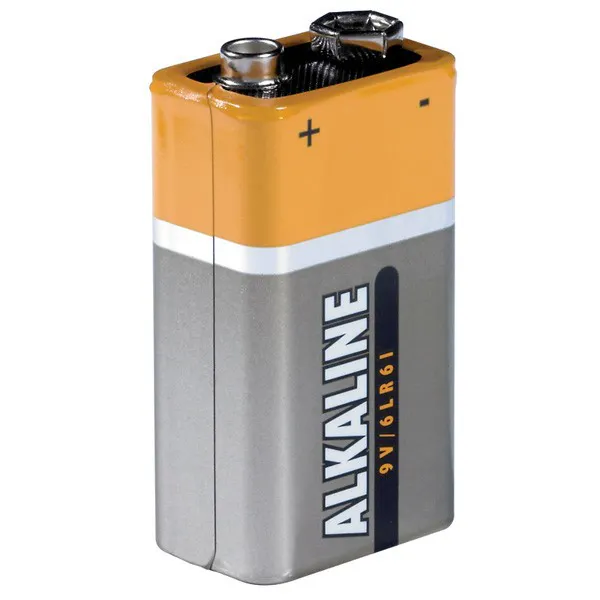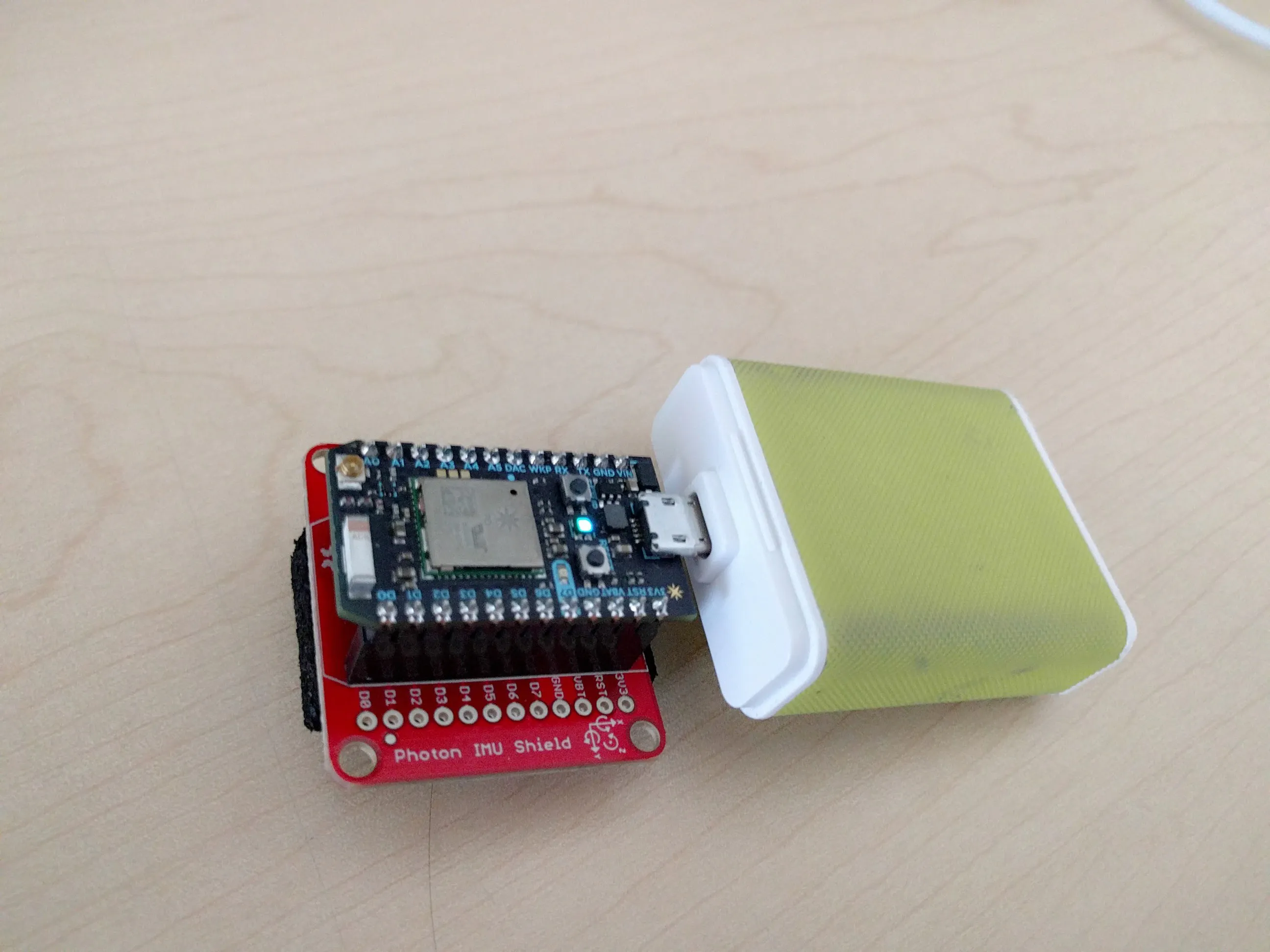# How to Impress Darth Vader

I want to impress Darth Vader with this Photon/Littlebits project. I tried different things, but only way is to use the Force.

IntermediateFull instructions provided4 hours1,295

## Things used in this project

### Hardware componentsParticle Photon
×1
 littleBits bend sensor
×1
 sparkfun photon imu
×1
 glove
×1
 usb power bank
×1
 littleBits cloudBit
×1×1
×1
 glove
×19V battery (generic)
×1

### Software apps and online services

 Vuforia
 Littlebits Cloudbit
 Particle IDEUnity

## Schematics

### As Shown

Just the IMU, Photon and USB power bank.## Code

### LittlebitsWebSocket.cs

C#
This one is to communicate Unity3d with Littlebits CloudBit via Websockets
```using UnityEngine;
using System.Collections;
using System;
using System.Security.Policy;
using Newtonsoft.Json;
using Newtonsoft.Json.Linq;
using WebSocketSharp;
using WebSocketSharp.Net;

public class LittlebitsWebSocket : MonoBehaviour {

public string device_id = "<Device ID Here>";
private string uri = "wss://api-stream.littlebitscloud.cc/primus/?access_token=";
public int value;
WebSocket ws;
GameObject energyLight;
EnergyScript energyScript;
// Use this for initialization
void Start () {
uri = uri + access_token;

energyLight = GameObject.FindGameObjectWithTag ("Light");
energyScript = energyLight.GetComponent<EnergyScript> ();
init ();
}

// Update is called once per frame
void Update () {

}

private void init()
{
}

{
ws = new WebSocket (uri);
{
// Set the WebSocket events.

//connect to littlebits and subscribe to a device
ws.OnOpen += (sender, e) =>
{
var data = new JObject();
var args1 = new JObject();

ws.Send(data.ToString());
};

// when we receive a message from littlebits, we need to parse the data
ws.OnMessage += (sender, e) =>
{
var data = e.Data.Substring(1,e.Data.Length -2);
var newData = data.Replace("\\", "");
JObject json = JObject.Parse(newData);
{
//if (value != newValue)
{
energyScript.LocalVelocity_Y = value / 10;
}
value = newValue;
Debug.Log ("Cloudbit:" + value);
}
};

ws.OnError += (sender, e) =>
Debug.Log ("Error >>" + e.Message);

ws.OnClose += (sender, e) =>
Debug.Log(String.Format("WebSocket Close ({0})", e.Code));

#if DEBUG
// To change the logging level.
ws.Log.Level = LogLevel.Trace;

// To change the wait time for the response to the Ping or Close.
ws.WaitTime = TimeSpan.FromSeconds(10);

// To emit a WebSocket.OnMessage event when receives a ping.
ws.EmitOnPing = true;
#endif
}
ws.ConnectAsync ();
}
}
```

### PhotonWS.cs

C#
This is what I used to communicate Photon with Unity3d App via UDP sockets
```using UnityEngine;
using System.Collections;
using System;
using System.Security.Policy;
using Newtonsoft.Json;
using Newtonsoft.Json.Linq;
using System.Collections.Generic;
using System.Net.Sockets;
using System.Net;
using System.Text;

public class PhotonWS : MonoBehaviour {

UdpClient client;
int port = 33333;

public float Value;

public GameObject Light;
// Use this for initialization
void Start () {
init ();
}

private List<float> initialSizes = new List<float>();

void Awake() {
// Save off all the initial scale values at start.
ParticleSystem[] particles = Light.GetComponentsInChildren<ParticleSystem>();
foreach (ParticleSystem particle in particles) {
ParticleSystemRenderer renderer = particle.GetComponent<ParticleSystemRenderer>();
if (renderer) {
}
}
}

public void Update() {
}

// Scale all the particle components based on parent.
int arrayIndex = 0;
ParticleSystem[] particles = Light.GetComponentsInChildren<ParticleSystem>();
foreach (ParticleSystem particle in particles) {
particle.startSize = initialSizes[arrayIndex++] * (value / 10);
ParticleSystemRenderer renderer = particle.GetComponent<ParticleSystemRenderer>();
if (renderer) {
renderer.lengthScale = initialSizes[arrayIndex++] *
Light.transform.localScale.magnitude;
renderer.velocityScale = initialSizes[arrayIndex++] *
Light.transform.localScale.magnitude;
}
}
}

private void init()
{

// ----------------------------
// Abhören
// ----------------------------
// Lokalen Endpunkt definieren (wo Nachrichten empfangen werden).
// Einen neuen Thread für den Empfang eingehender Nachrichten erstellen.
}

{
client = new UdpClient(port);
while (true)
{

try
{
IPEndPoint anyIP = new IPEndPoint(IPAddress.Any, 0);

string text = Encoding.UTF8.GetString(data);

//var n = text.Split('A');
//print(">> " + n);
// latest UDPpacket

Value = Convert.ToSingle (rot );

}
catch (Exception err)
{
print(err.ToString());
}
}

}
}
```

### imu-udp.ino

Arduino
This is what I used to flash Arduino, so it can communicate with Unity3d via UDP sockets
```// This #include statement was automatically added by the Particle IDE.
#include "Kalman/Kalman.h"

#include "SparkFunLSM9DS1/SparkFunLSM9DS1.h"
#include "math.h"

//////////////////////////
// LSM9DS1 Library Init //
//////////////////////////
// Use the LSM9DS1 class to create an object. [imu] can be
// named anything, we'll refer to that throught the sketch.
LSM9DS1 imu;

///////////////////////
// Example I2C Setup //
///////////////////////
// SDO_XM and SDO_G are both pulled high, so our addresses are:
#define LSM9DS1_M   0x1E // Would be 0x1C if SDO_M is LOW
#define LSM9DS1_AG  0x6B // Would be 0x6A if SDO_AG is LOW

////////////////////////////
// Sketch Output Settings //
////////////////////////////
#define PRINT_CALCULATED
//#define PRINT_RAW
#define PRINT_SPEED 10 // 250 ms between prints

// Earth's magnetic field varies by location. Add or subtract
// a declination to get a more accurate heading. Calculate
// your's here:
// http://www.ngdc.noaa.gov/geomag-web/#declination
//#define DECLINATION -8.58 // Declination (degrees) in Boulder, CO.

#define DECLINATION -3.42 // Declination (degrees) in Fort Worth.

int i = 0;
// An UDP instance to let us send and receive packets over UDP
UDP Udp;

uint8_t server[] = { 192, 168, 43, 1}; // ip address of my phone

unsigned int localPort = 33333;

uint32_t timer;

void setup()
{

Serial.begin(115200);

sprintf(myIpAddress, "%d.%d.%d.%d", myIp, myIp, myIp, myIp);

// start the UDP
Udp.begin(localPort);

// Before initializing the IMU, there are a few settings
// we may need to adjust. Use the settings struct to set
// the device's communication mode and addresses:
imu.settings.device.commInterface = IMU_MODE_I2C;
// The above lines will only take effect AFTER calling
// imu.begin(), which verifies communication with the IMU
// and turns it on.
if (!imu.begin())
{
Serial.println("Failed to communicate with LSM9DS1.");
Serial.println("Double-check wiring.");
Serial.println("Default settings in this sketch will " \
"work for an out of the box LSM9DS1 " \
"Breakout, but may need to be modified " \
"if the board jumpers are.");
while (1)
;
}

timer = micros();

}

void loop()
{

printAttitude(imu.ax, imu.ay, imu.az, -imu.my, -imu.mx, imu.mz, imu.gx, imu.gy, imu.gz);

//printGyro();

delay(PRINT_SPEED);
}

void printGyro()
{
// To read from the gyroscope, you must first call the
// readGyro() function. When this exits, it'll update the
// gx, gy, and gz variables with the most current data.

// Now we can use the gx, gy, and gz variables as we please.
// Either print them as raw ADC values, or calculated in DPS.
Serial.print("G: ");
#ifdef PRINT_CALCULATED
// If you want to print calculated values, you can use the
// calcGyro helper function to convert a raw ADC value to
// DPS. Give the function the value that you want to convert.
Serial.print(imu.calcGyro(imu.gx), 2);
Serial.print(", ");
Serial.print(imu.calcGyro(imu.gy), 2);
Serial.print(", ");
Serial.print(imu.calcGyro(imu.gz), 2);
Serial.println(" deg/s");
#elif defined PRINT_RAW
Serial.print(imu.gx);
Serial.print(", ");
Serial.print(imu.gy);
Serial.print(", ");
Serial.println(imu.gz);
#endif

Udp.beginPacket(IPfromBytes, localPort);

Udp.endPacket();
}

void printAccel()
{
// To read from the accelerometer, you must first call the
// readAccel() function. When this exits, it'll update the
// ax, ay, and az variables with the most current data.

// Now we can use the ax, ay, and az variables as we please.
// Either print them as raw ADC values, or calculated in g's.
Serial.print("A: ");
#ifdef PRINT_CALCULATED
// If you want to print calculated values, you can use the
// calcAccel helper function to convert a raw ADC value to
// g's. Give the function the value that you want to convert.
Serial.print(imu.calcAccel(imu.ax), 2);
Serial.print(", ");
Serial.print(imu.calcAccel(imu.ay), 2);
Serial.print(", ");
Serial.print(imu.calcAccel(imu.az), 2);
Serial.println(" g");
#elif defined PRINT_RAW
Serial.print(imu.ax);
Serial.print(", ");
Serial.print(imu.ay);
Serial.print(", ");
Serial.println(imu.az);
#endif

}

Kalman kalmanX; // Create the Kalman instances
Kalman kalmanY;
double gyroXangle, gyroYangle; // Angle calculate using the gyro only
double compAngleX, compAngleY; // Calculated angle using a complementary filter
double kalAngleX, kalAngleY; // Calculated angle using a Kalman filter

// Calculate pitch, roll, and heading.
// Pitch/roll calculations take from this app note:
// http://cache.freescale.com/files/sensors/doc/app_note/AN3461.pdf?fpsp=1
// Heading calculations taken from this app note:
void printAttitude(
float ax, float ay, float az, float mx, float my, float mz, float gx, float gy, float gz)
{
float roll = atan2(ay, az);
float pitch = atan2(-ax, sqrt(ay * ay + az * az));

if (my == 0)
heading = (mx < 0) ? 180.0 : 0;
else

heading -= DECLINATION * M_PI / 180;

double dt = (double)(micros() - timer) / 1000000; // Calculate delta time
timer = micros();

// Convert everything from radians to degrees:
pitch *= 180.0 / M_PI;
roll  *= 180.0 / M_PI;

double gyroXrate = gx / 131.0; // Convert to deg/s
double gyroYrate = gy / 131.0; // Convert to deg/s

if ((pitch < -90 && kalAngleY > 90) || (pitch > 90 && kalAngleY < -90)) {
kalmanY.setAngle(pitch);
compAngleY = pitch;
kalAngleY = pitch;
gyroYangle = pitch;
} else
kalAngleY = kalmanY.getAngle(pitch, gyroYrate, dt); // Calculate the angle using a Kalman filter

if (abs(kalAngleY) > 90)
gyroXrate = -gyroXrate; // Invert rate, so it fits the restriced accelerometer reading
kalAngleX = kalmanX.getAngle(roll, gyroXrate, dt); // Calculate the angle using a Kalman filter

double accelX = imu.calcAccel(ax);
double accelY = imu.calcAccel(ay);
double accelZ = imu.calcAccel(az);

Udp.beginPacket(IPfromBytes, localPort);

Udp.endPacket();